# ggtricks

A package to create sector charts easily using Cartesian coordinates.

R
ggplot2
Dataviz
Tidyverse
Author

Abdoul ISSA BIDA

Published

June 6, 2023

Welcome data folks. Today, we walk through a new grammar of graphics extension package.

Yeah, I know, you should be overwhelmed with all the `ggplot2` package extensions out there, but I promise this one, like all the others, comes with its own set of features, so you won’t be disappointed.

## Motivation

For my short experience (3-4 years) with the {tidyverse} ecosystem, and particularly with the grammar of graphics philosophy, I was surprised that there was no native function for making sector charts, de facto using Cartesian coordinates.

There were methods to derive bar charts to sectors one, using polar coordinates, I’ve applied them a lot in the past, but usually ended up frustated to not be able to easily add labels to the plot using the Catersian system, in which I best project myself.

So, a few months ago, I decided to create a package to fix this problem.
If sectors charts rhyme with pie and donut charts, it is a kind a chart not used enough in my opinion, which pushed me to immerse myself in the package, the series of circles chart.

## `geom_series_circles`

I like books. I like old style. And I like data visualization.
So, you won’t be surprised that I usually find myself stuck with data visualization books from the early 1900s. That’s how, I was drawn to this chart featured in Charts and Graphs by Karl G. Karslen.

According to the book:

It consists in using whole circles and fractions of fragments of circles, all of uniform radii.

This type of chart can be a good alternative for presenting information in the form of a bar chart, or financial or proportional data, in which the circle can be taken to represent dollars (or something) and the fractions of circles parts of dollars (or parts of something). I decide to call the function drawing this kind of chart `geom_series_circles`.

Let’s use the example data below to illustrate the use of the function.

Data code
``````library(tidyverse)
prices_index <- tribble(
~article, ~index,
"All articles", 219,
"Plate beef", 187,
"Chuck roast", 186 ,
"Bacon", 216 ,
"Lard", 266 ,
"Cheese", 197 ,
"Butter", 204 ,
"Coffee", 165,
"Hens", 224,
"Rice", 215 ,
"Flour", 267 ,
"Potatoes", 606 ,
"Sugar", 485 ,
"Sirloin steak", 192 ,
"Round steak", 202 ,
"Rib roast", 181 ,
"Corn meal", 233,
"Tea", 137 ,
"Ham", 224 ,
"Pork chops", 238 ,
"Milk", 194 ,
"Eggs", 268
)``````

Let’s install & load the package.

``````install.packages("ggtricks")

For other installation options, including the development version, check the package directory on github: https://github.com/AbdoulMa/ggtricks.

Let’s plot now.

``````  theme_post <- function(...) {
theme_minimal() +
theme(
text = element_text(family = "Atkinson Hyperlegible"),
axis.text = element_text(color = "black"),
panel.grid = element_blank(),
plot.background = element_rect(fill = "white", color = NA),
...
)
}
prices_index |>
mutate(
article = fct_rev(fct_inorder(article)),
# divide the index /100
# to represent each 100 index by 1 circle
# otherwise the result would not be visually pleasing
index = index /100
) |>
ggplot() +
geom_series_circles(aes(
# the indexes will represented horizontally
# and articles vertically
# Feel free to inverse x and y if you want the opposite
x = index,
y =  article
)) +
coord_equal(clip = "off") +
theme_post()``````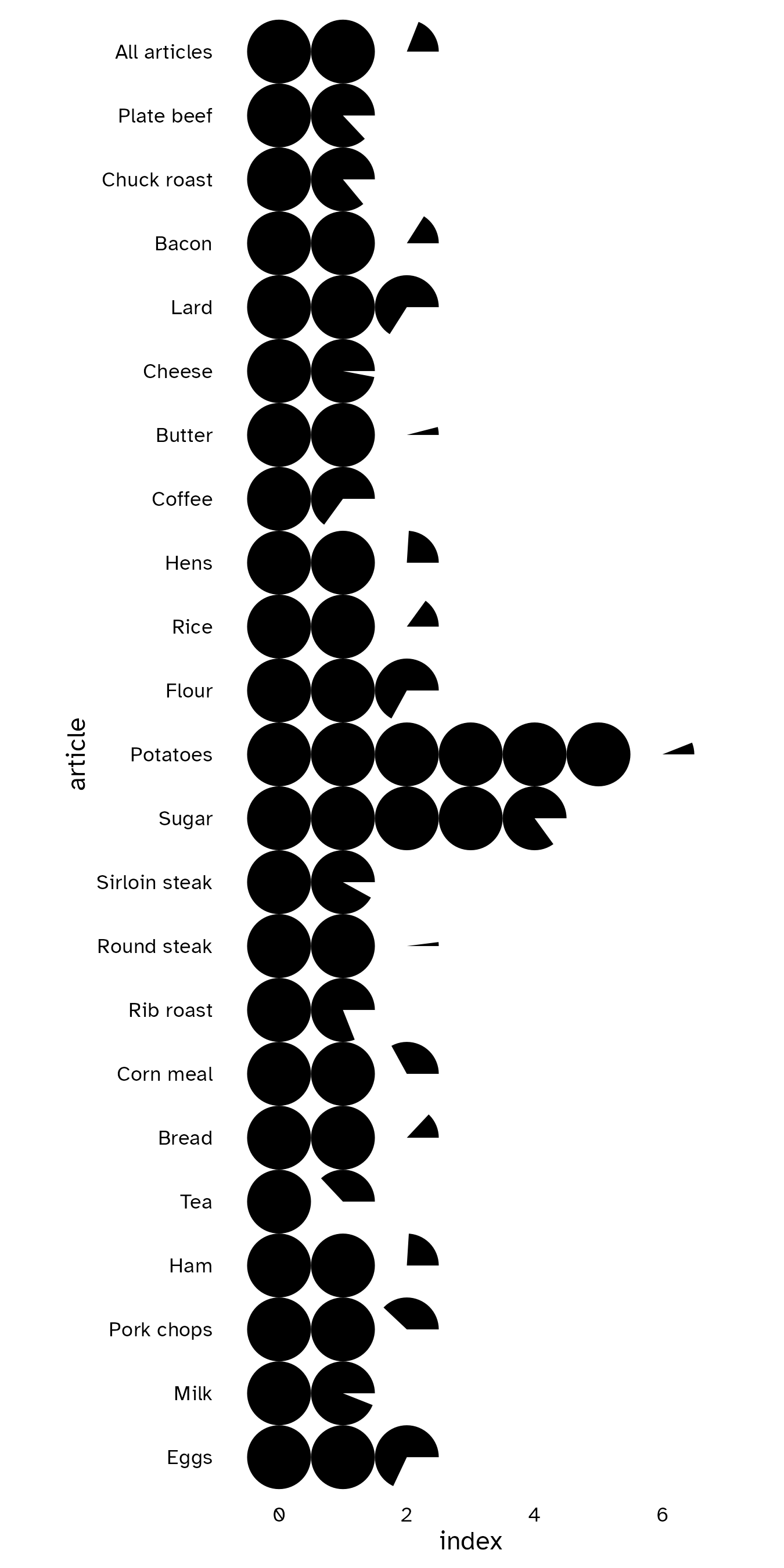That’s it. We have our series of circles, where each complete circle represents `100` of food index, and each fragment of circle represents a value proportional to `100`.

As `{ggplot2}` aficionados, I know you will ask for customization options.
So I add a native `fill` argument in the `aes()` mapping function.
You also have the`color` and `linewidth` option arguments to drive the color and width of circle borders respectively.

``````prices_index |>
mutate(
article = fct_rev(fct_inorder(article)),
index = index /100
) |>
ggplot() +
geom_series_circles(aes(
x = index,
y =  article,
fill = article
),
color = "black",
linewidth = 1.85
) +
coord_equal(clip = "off") +
theme_post(
legend.position = "none",
axis.title = element_blank(),
axis.text.x = element_blank(),
axis.text.y = element_text(face = "bold", color = "black", size = rel(1.25))
)``````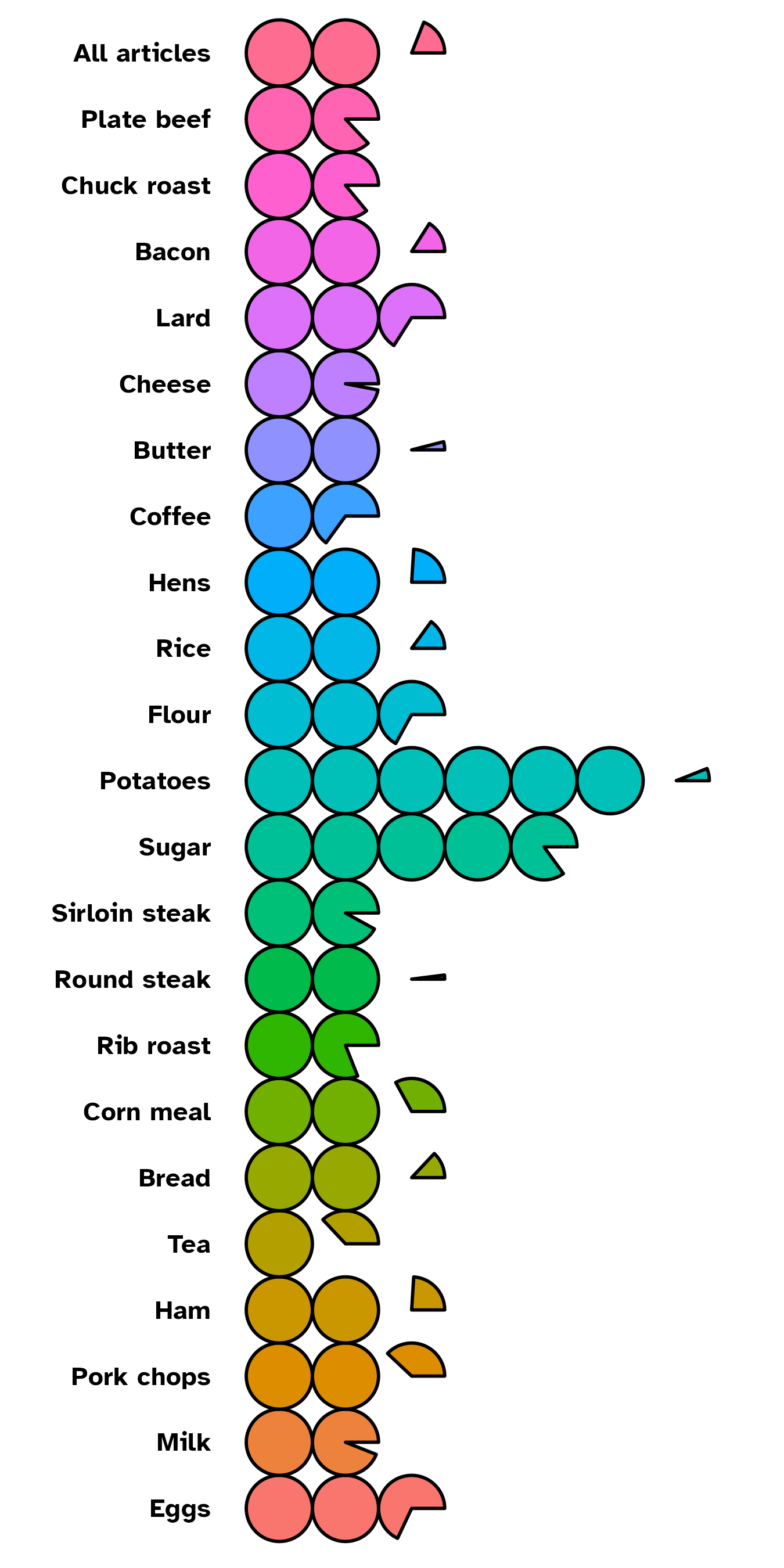## `geom_series_text`

Of course, you can choose, to customize the categories labels by setting `axis.text` in `theme_*()` function. But the need may come to add labels at series of circles boundary positions.
There comes `geom_series_text()` function. See it as a companion function to `geom_series_circles()`.

``````prices_index |>
mutate(
article = fct_rev(fct_inorder(article)),
index = index /100
) |>
ggplot() +
geom_series_circles(aes(
x = index,
y =  article,
fill = article
),
color = "black",
linewidth = 1.85
) +
geom_series_text(
aes(
x = index,
y = article,
label = index
),
size = 3.5,
family = "Atkinson Hyperlegible",
fontface = "bold",
hjust = 0
) +
coord_equal(clip = "off") +
theme_post(
legend.position = "none",
axis.title = element_blank(),
axis.text.x = element_blank(),
axis.text.y = element_text(family = "Atkinson Hyperlegible", face = "bold", color = "black", size = rel(1.25))
)``````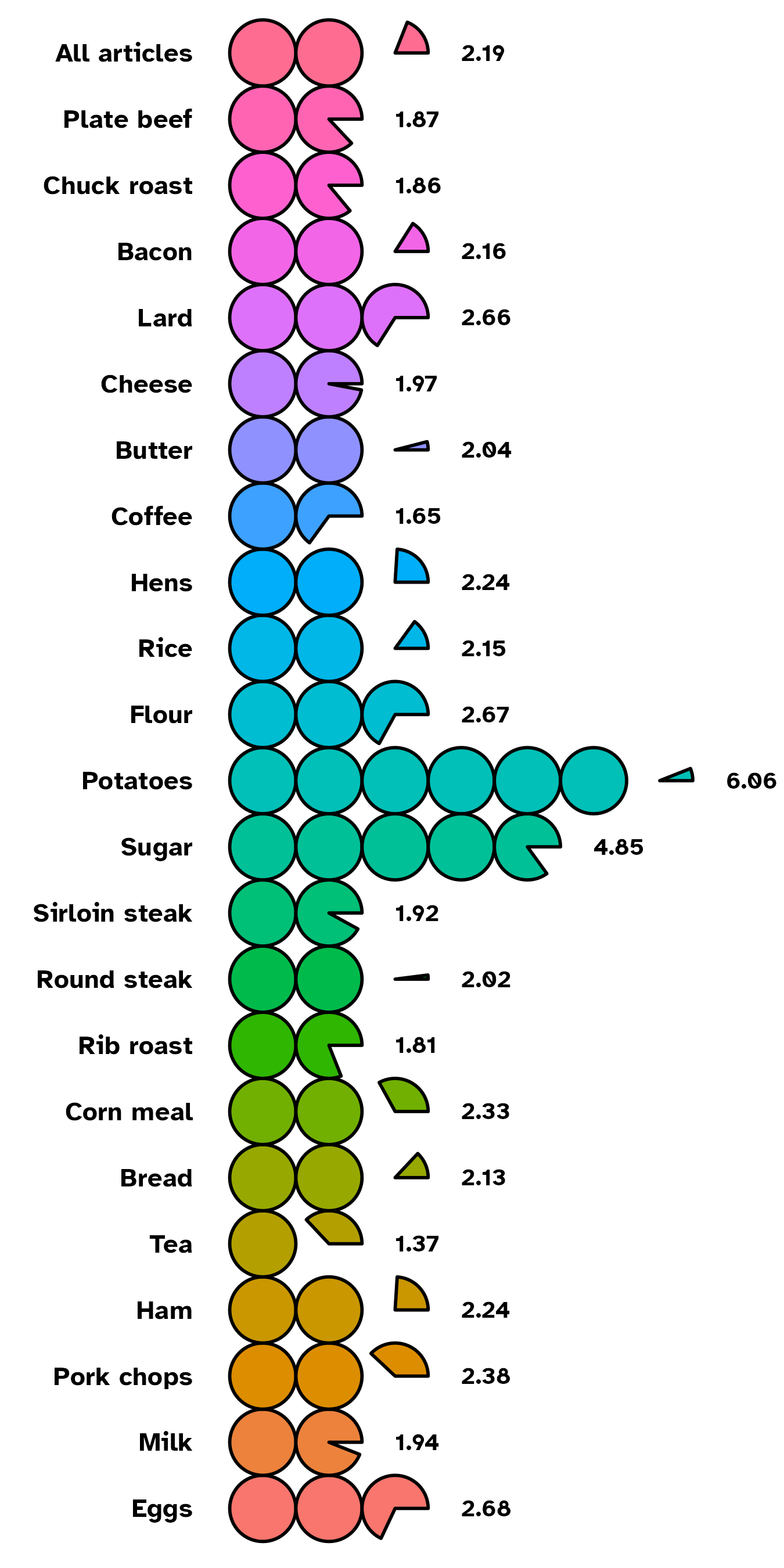For many more options (starting angle, circle radius … etc), for both functions, `?ggtricks::geom_series_circles` and `?ggtricks::geom_series_text` in your console will open the help window with documentations.

So it’s time to dive in creating more classic sector charts with `ggtricks`.

## `geom_pie`

As mentioned, when starting this blog post, there had always been ways to create sector charts with `ggplot2` using `coord_polar` with pros and cons when adding labels. So before deciding to implement the `geom_pie` function, I searched for possible solutions, but I didn’t find one that met my need (except the base R graphics pie function ).
That’s where the package developer in me came in!

Let’s use an example data set.

Data Code
``````my_df <- data.frame(
cat = paste0("Prod ", 1:4),
val = c(87, 34, 21, 8)
)

categories_fills <- c(
"Prod 1" = "#3E71EC",
"Prod 2" = "#A9A9A9",
"Prod 3" = "#7942A6",
"Prod 4" = "#F7324B"
)``````
``````my_df |>
ggplot() +
geom_pie(aes(cat = cat, val = val, fill = cat),
) +
coord_equal() +
scale_fill_manual(
values = categories_fills
) +
theme_post()``````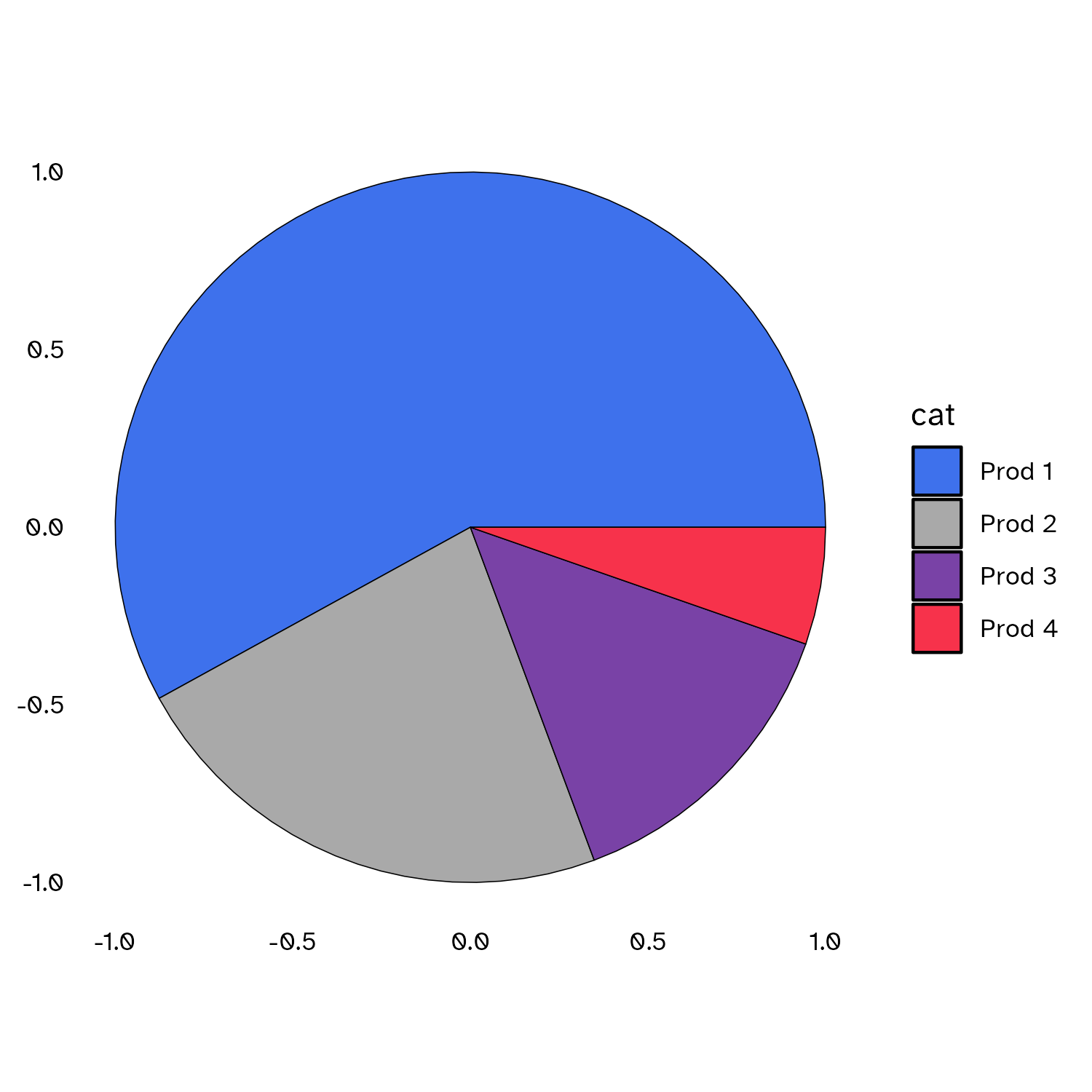Well, we have the pie in Cartesian system coordinates. To prove it, let’s place `René Descartes` at position `(-0.5, -0.5)`.

``````my_df |>
ggplot() +
geom_pie(aes(cat = cat, val = val, fill = cat),
) +
annotate(ggplot2::GeomText, x = -0.5, y = -0.5, label = "René\n DESCARTES", family = "Atkinson Hyperlegible") +
coord_equal() +
scale_fill_manual(
values = categories_fills
) +
theme_post()``````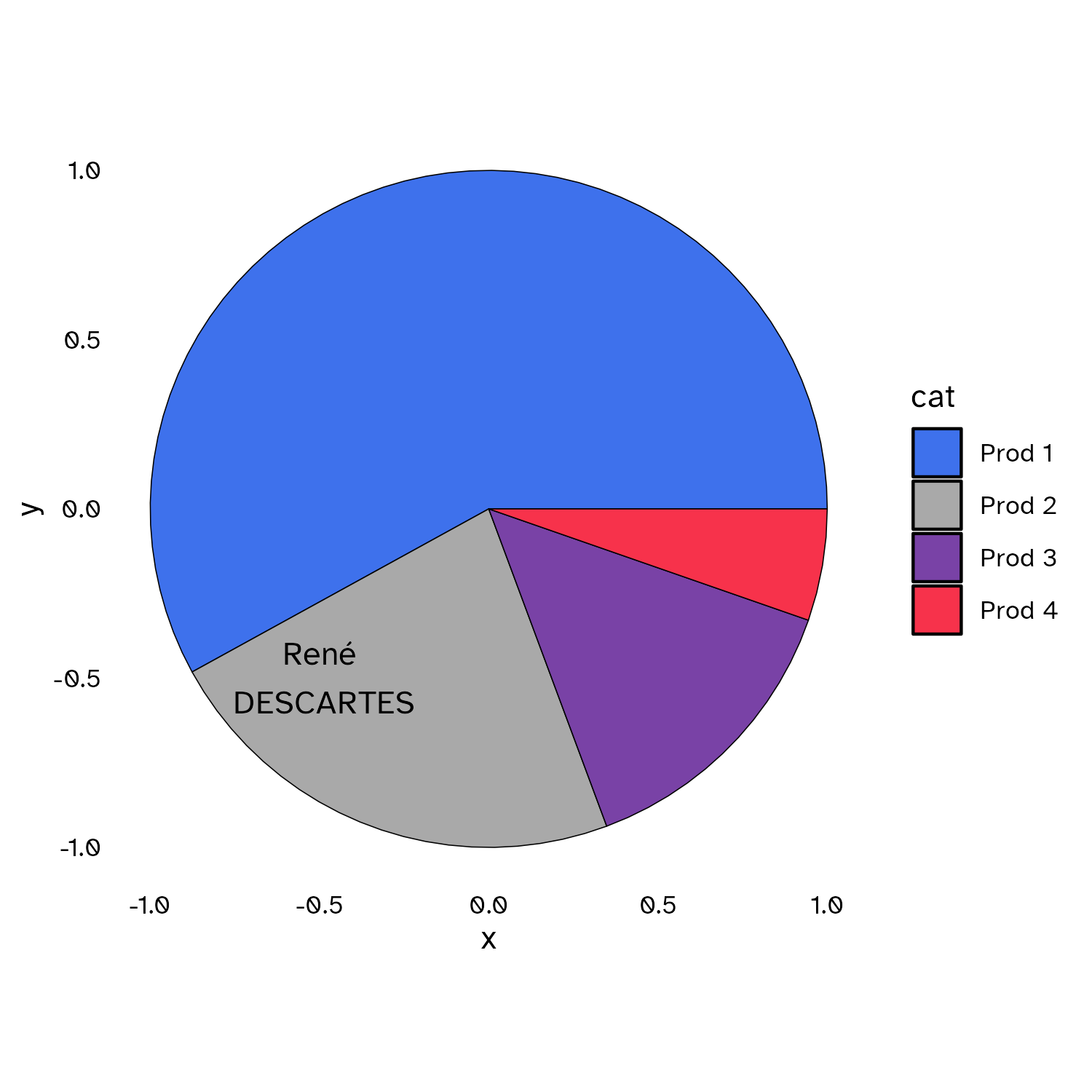There are many customization options:

• `init_angle` to set the starting angle of your pie.
``````my_df |>
ggplot() +
geom_pie(aes(cat = cat, val = val, fill = cat),
init_angle = 0
# init_angle = 60,
# init_angle = 120,
# init_angle = 180
) +
coord_equal() +
scale_fill_manual(
values = categories_fills
) +
theme_minimal()``````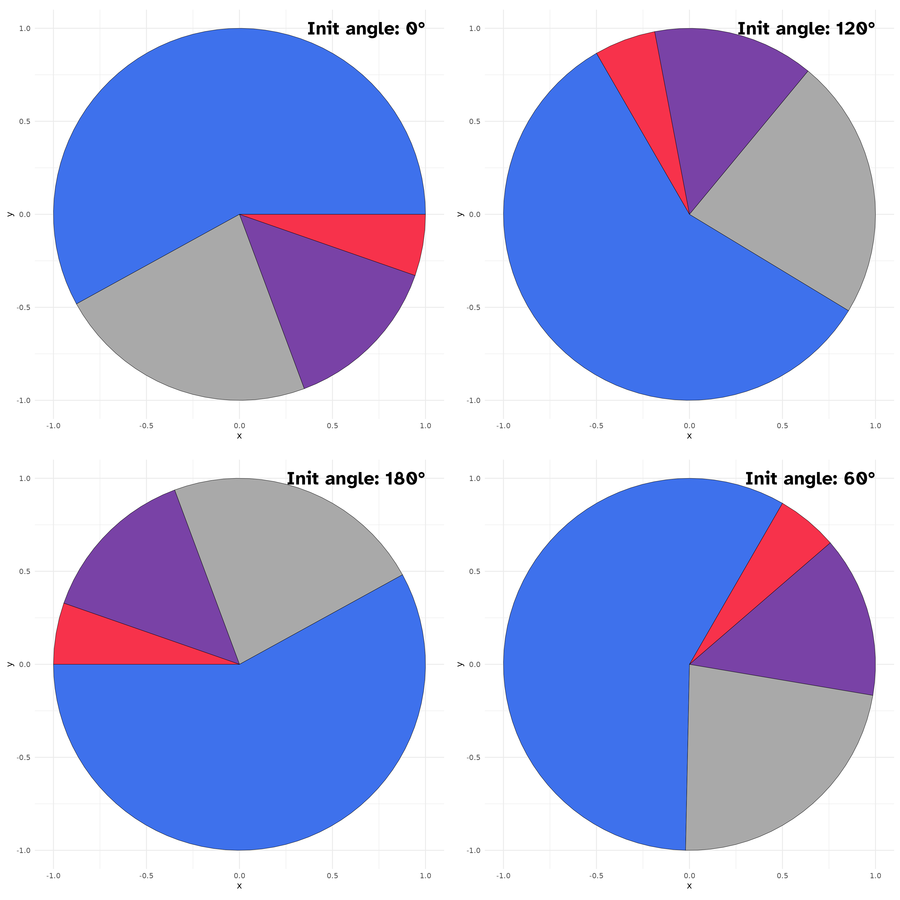• `spotlight_max` & `spotlight_position`

If you want the category with the max value to determine the slices positions, you can set the `spotlight_max` parameter to `true`. Then the category with the max value will be placed at `spotlight_position` (by default `top`, others possible values are: `right`, `bottom` and `left.`)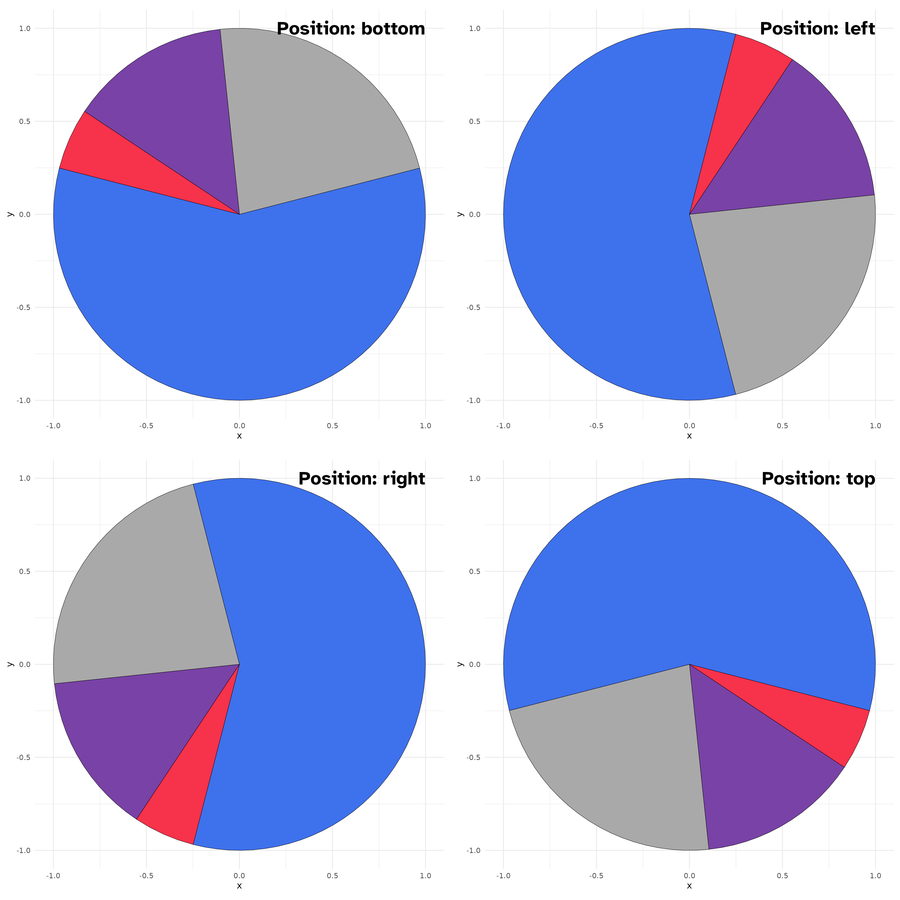• `spotlight_cat`

Maybe, you want a specific category to drive the slices positions rather than the category with the maximum value?

This is where comes the `spotlight_cat` parameter to define the driving category.
Also here, you can combine the `spotlight_cat` parameter value with `spotlight_position` to specify its position.

``````my_df |>
ggplot() +
geom_pie(aes(cat = cat, val = val, fill = cat),
spotlight_cat = "Prod 1",
spotlight_position = "top"
) +
coord_equal() +
scale_fill_manual(
values = categories_fills,
guide = "none"
) +
theme_minimal()``````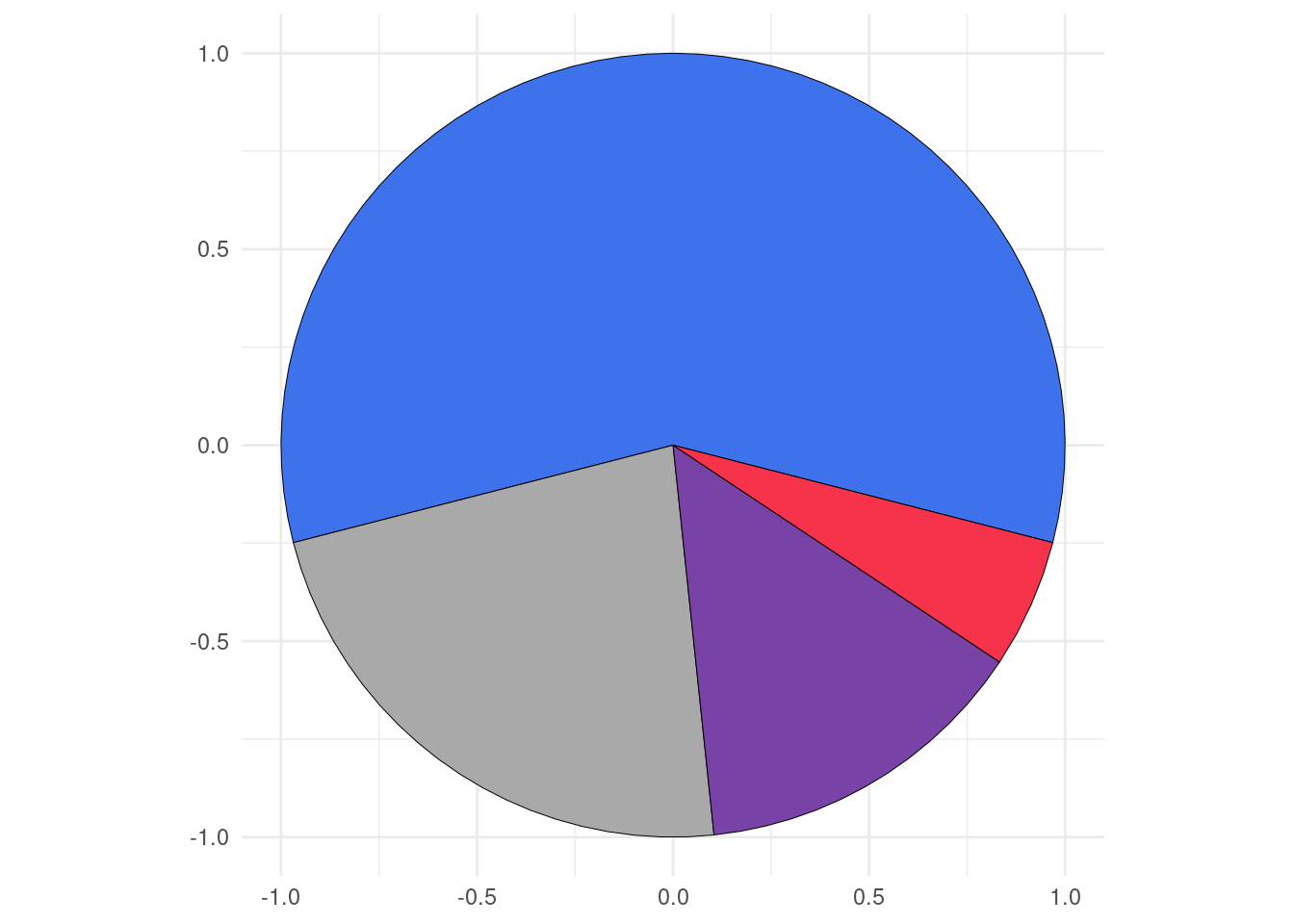• `labels`

As I know that it can be difficult to know the coordinates of the center positions of the category slices, I define a default `label` mapping which will place the provided labels at this position.
When `label` mapping is defined, you can set the `labels_with_tick` parameter to `TRUE` to add tick marks to the centers positions of the slices.

``````my_df |>
ggplot() +
geom_pie(
aes(cat = cat, val = val, fill = cat, label = cat)
# labels_with_ticks = TRUE
) +
coord_equal() +
scale_fill_manual(
values = categories_fills,
guide = "none"
) +
theme_minimal()``````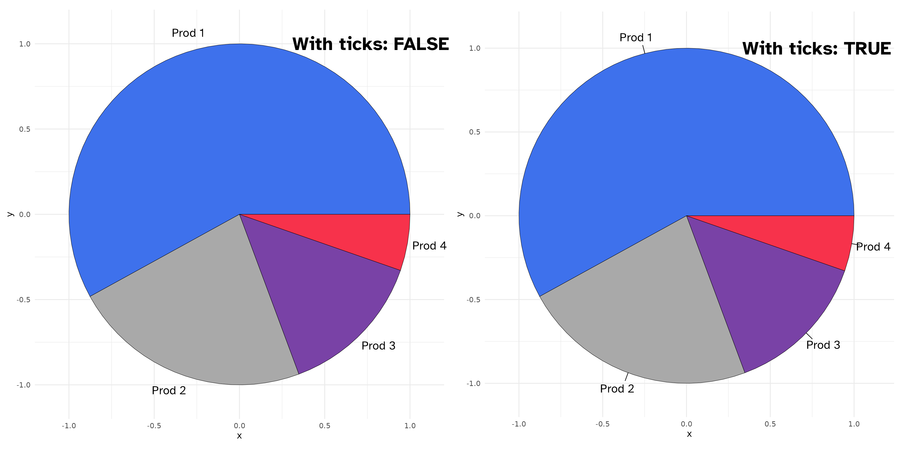## `geom_donut`

Donut is just pie with a hole in it.
There are two parameters `r1` and `r2` to define the thickness of the donut.

``````my_df |>
ggplot() +
geom_donut(aes(cat = cat, val = val, fill = cat),
r1 = 1, r2 = .65
# r1 =  1 , r2 = .35
) +
coord_equal() +
scale_fill_manual(
values = categories_fills,
guide = "none"
) +
theme_minimal()``````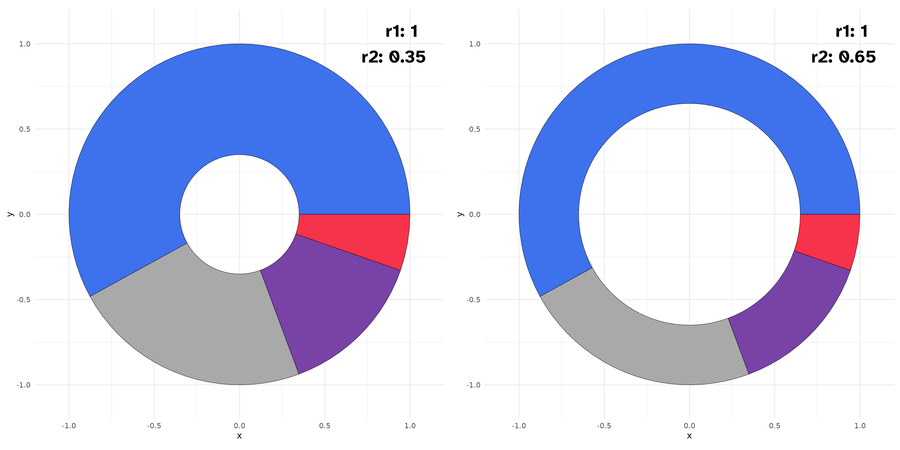All others parameters available for `geom_pie` are also available here.

## `geom_slice`

It is a portion of pie, by default a half (180 deg). You can set the `slice_angle` portion as needed.

``````my_df |>
ggplot() +
geom_slice(aes(cat = cat, val = val, fill = cat),
slice_angle = 180 # ,
# slice_angle = 120
) +
coord_equal() +
scale_fill_manual(
values = categories_fills,
guide = "none"
) +
theme_minimal()``````

Also here, you can set the starting angle position with `init_angle`. Note here that there are no `spotlight_max`, `spotlight_cat` parameters, since we are not drawing a complete circle (but theoretically you can, if you set `slice_angle` to 360, which means a pie.)

``````my_df |>
ggplot() +
geom_slice(aes(cat = cat, val = val, fill = cat),
init_angle = 30 # ,
# init_angle = 90
) +
coord_equal() +
scale_fill_manual(
values = categories_fills,
guide = "none"
) +
theme_minimal()``````

You can however set the slice position with `slice_position` (possible values are: `top`, `right`, `bottom`, and `left`). Soon, I will post more detailed examples on the package website: https://abdoulma.github.io/ggtricks/.

## `geom_donut_slice`

It is a slice of donut plot. As a `geom_donut`, it is driven by 2 radii and as a slice plot, it has a defined slice angle.

``````my_df |>
ggplot() +
geom_donut_slice(aes(cat = cat, val = val, fill = cat),
r1 = 1, r2 = .65
# r1 = 1, r2  = .35,
# slice_angle = 90
# slice_angle = 120
# slice_angle = 180
) +
coord_equal() +
scale_fill_manual(
values = categories_fills,
guide = "none"
) +
theme_minimal()``````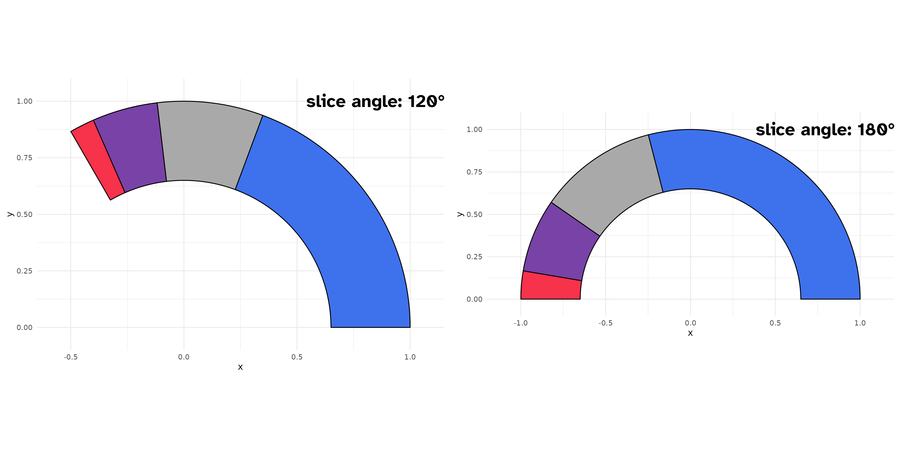`geom_donut_slice` also has special parameter `link_with_origin`, if you want to connect the donut slice boundaries with origin.

``````my_df |>
ggplot() +
geom_donut_slice(aes(cat = cat, val = val, fill = cat),
r1 = 1, r2 = .65,
slice_angle = 120,
slice_position = "top",
) +
coord_equal(clip = "off") +
scale_fill_manual(
values = categories_fills,
guide = "none"
) +
theme_minimal()``````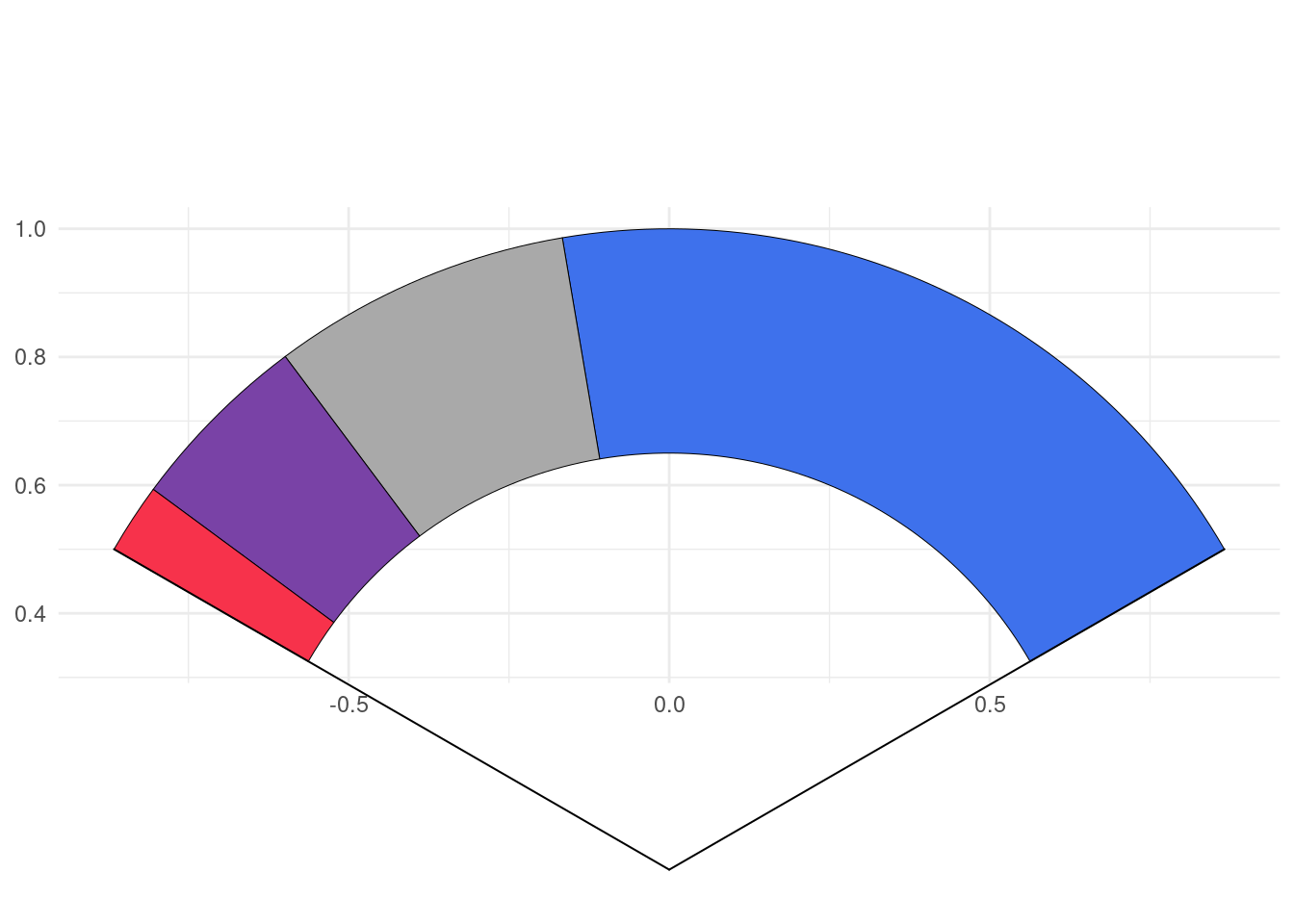## Limitations

As you might have noticed, to generate circle, I use `coord_equal()`, using `coord_cartesian()` will zoom the plot, not generating a appealing circle shape even if the underlying drawn plot is a circle. So, we fix, the `aspect ratio` to force :

the physical representation of data units on the axes.

according to the official documentation. Of course, you shouldn’t edit the default `ratio = 1` that ensures that one unit on `x-axis` is the same length as one unit on the `y-axis`.

When using `geom_series_circles()`, the desire will come one day to combine it with `facet_wrap()` or `facet_grid()` or any faceting function, you should not, or not the way you envision.

Since we are using `coord_equal()`, you won’t be able to set `scales` parameter, which I strongly suspect you to try to do. So for the moment, I advise you not to do so. However, I will provide some tips to go through those restrictions on package website https://abdoulma.github.io/ggtricks/. If you have any specific questions or suggestions, do not hesitate to reach out to me.

## Future improvements

In the coming weeks, additional features will be added to current geoms:

• Detach spotlighted category
• Variate radii for the representation of categories
• Label displaying in mapping (choose categories we want to display)
• Special key draw for pie and slice and another one for `donut` and `donut_slice`.

As announced at start, I am not limiting the package to sector charts, so additional `geom` styles will be added, and if you have suppositions, fee free to open an issue, I am open to all contributions.

## Citation

BibTeX citation:
``````@online{issabida2023,
author = {Abdoul ISSA BIDA},
title = {Ggtricks},
date = {2023-06-06},
url = {https://www.abdoulblog.com},
langid = {en}
}
``````